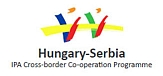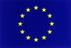## Mathematica and Geogebra and parametric functions

Kulcsszavak: ICT, Wolfram Mathematica, Geogebra, functions, parameters, visualization
Absztrakt:

#### Full title: The impact of dynamic properties of the software packages Mathematica and GeoGebra to the examining and graphing of functions with parameters

Examining and graphing functions in GeoGebra and Wolfram Mathematica environment is presented. The students are supposed to determine the domain, zeros, sign, derivate, monotonicity, extreme points, inflection points, convexity and, finally, the graph of the function. The packages GeoGebra and Mathematica enable students to consider the influence of parameters on function characteristics.

The development is co-financed by the European Union in the frame of the project IPA HU-SRB/1203/221/024: Non-Standard Forms of Teaching Mathematics and PhysicsSzükséges szoftverek:

Wolfram Mathematica (CDF Player)

Geogebra

Tartalomjegyzék: